﻿ Yield to maturity: What is it, Calculation, Uses, Importance, FAQ | Poems

# Yield to maturity

## Yield to maturity

Investors should be aware that yield to maturity can be affected by changes in interest rates. If interest rates go up, the price of a bond will usually go down, and the yield to maturity will increase. Conversely, if interest rates go down, the price of a bond will usually go up, and the yield to maturity will decrease.

The yield to maturity is an important measure for bond investors, as it can give them an idea of the total return they can expect on their investment. It is also a useful tool for comparing different bonds.

## What is yield to maturity?

Yield to maturity measures the total return on an investment in a bond expressed as a percentage of the bond’s current market price. The yield to maturity accounts for both interest paid on the bond since purchase and any potential capital profits or losses.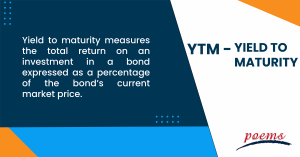## Understand yield to maturity

The rate of return an investor will receive if they keep a bond till it matures is known as yield to maturity. This rate takes into account the interest that the bond pays and any capital gains or losses that may occur as the bond’s price fluctuates.

When understanding yield to maturity, there are a few factors to remember. First, the yield to maturity is different from the coupon rate. The interest the bond pays out annually is the coupon rate, but the yield to maturity accounts for both interest payments and price fluctuations.

Second, the yield to maturity is a theoretical rate. An investor may not earn this exact rate if they sell the bond before it matures. Finally, the yield to maturity assumes that the bond will be held until it matures. The investor may receive a different rate of return if the bond is sold before it matures.

## How to calculate yield to maturity?

Bond returns are measured by yield to maturity. It is calculated by taking the interest payments on the bond and dividing them by the bond’s purchase price.

For example, if there’s a bond with a face value of 1,000 USD and an interest rate of 5%, the interest payments would be 50 USD per year. If the bond is purchased for 900 USD, the yield to maturity would be 5.56%.

## Uses of yield to maturity

Yield to maturity can be used to compare different bonds with different coupon rates and lengths of time until maturity. It can also be used to compare bonds with different market prices. For example, a bond with a lower market price will have a higher yield to maturity than a bond with a higher market price.

Bonds of various types can also be compared using yield to maturity. For example, a corporate bond usually has a higher yield to maturity than a government bond. This is because corporate bonds are considered riskier than government bonds.

Finally, you may purchase or sell a bond based on the yield to maturity. It may be a good idea to buy a bond if the yield to maturity is greater than the current market yield since that indicates that the bond is undervalued.

On the other hand, if the yield to maturity is lower than the current market yield, the bond is considered overvalued, and it may be a good time to sell the bond.

## Importance of yield to maturity

Yield to maturity is an important concept for bond investors to understand because it provides a way to compare the potential return of different bonds. Yield to maturity considers the bond’s current market price, the coupon payments, and the length of time until the bond matures. This makes it a good way to compare bonds of different prices, coupon rates, and maturities.

Remember that the yield to maturity is not the same as the current yield, which only looks at coupon payments. The yield to maturity also considers the bond’s price, which can fluctuate over time. This makes the yield to maturity a more accurate measure of the potential return on a bond.

Since the coupon rate has a set bond term for the whole year, this is the main distinction between the coupon rate and yield of maturity. The yield of maturity, on the other hand, varies according to different factors, including the number of years left until maturity and the current price for which the bond is sold.

The yield to maturity rates of a bond moves inversely with its price. Investors will expect higher returns as interest rates climb. As a result, bond prices will decline, which will inevitably cause the yield-to-maturity rate to increase.

The yield to maturity (YTM) is the total return expected from a bond if it is held until maturity. The coupon rate is simply the interest rate that the bond pays annually. The current yield is the YTM divided by the bond’s current market price.

To calculate the YTM, one must first determine the bond’s price. The YTM takes into account the price of the bond, the coupon payments, and the time to maturity. The current yield does not consider the bond price, only the coupon payments and time to maturity.

The YTM is always higher than the current yield because the current yield does not account for the bond’s price. The YTM is the most accurate measure of a bond’s return, while the current yield is a good measure of a bond’s interest rate.

It is possible for a bond’s yield to call to be higher than its yield to maturity. This can happen if the bond is callable at a price below its par value, and the yield to call is calculated using this call price. In this case, the yield to call will be higher than the yield to maturity since the yield to maturity is calculated using the bond’s par value.

To calculate yield to maturity on a trial error, determine the bond’s price using the trial and error method. Then, using the bond’s price and face value, calculate the yield to maturity.

## Category

### Read the Latest Market Journal#### Top traded counters in August 2023

Published on Sep 19, 2023 202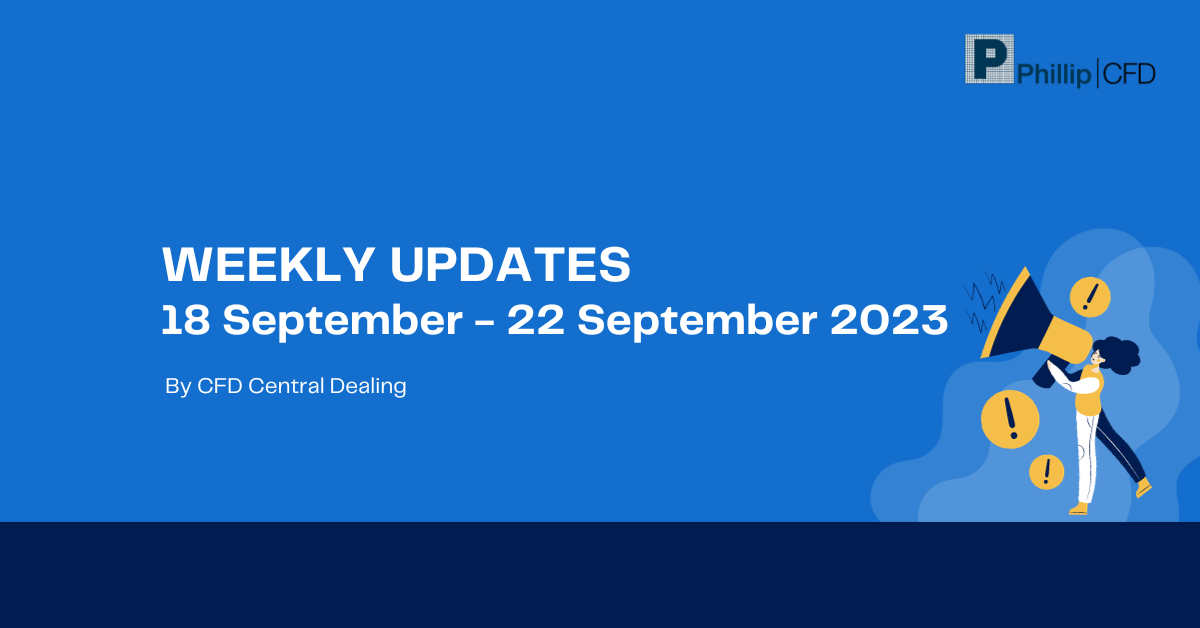#### Weekly Updates 18/9/23 – 22/9/23

Published on Sep 18, 2023 28

This weekly update is designed to help you stay informed and relate economic and company...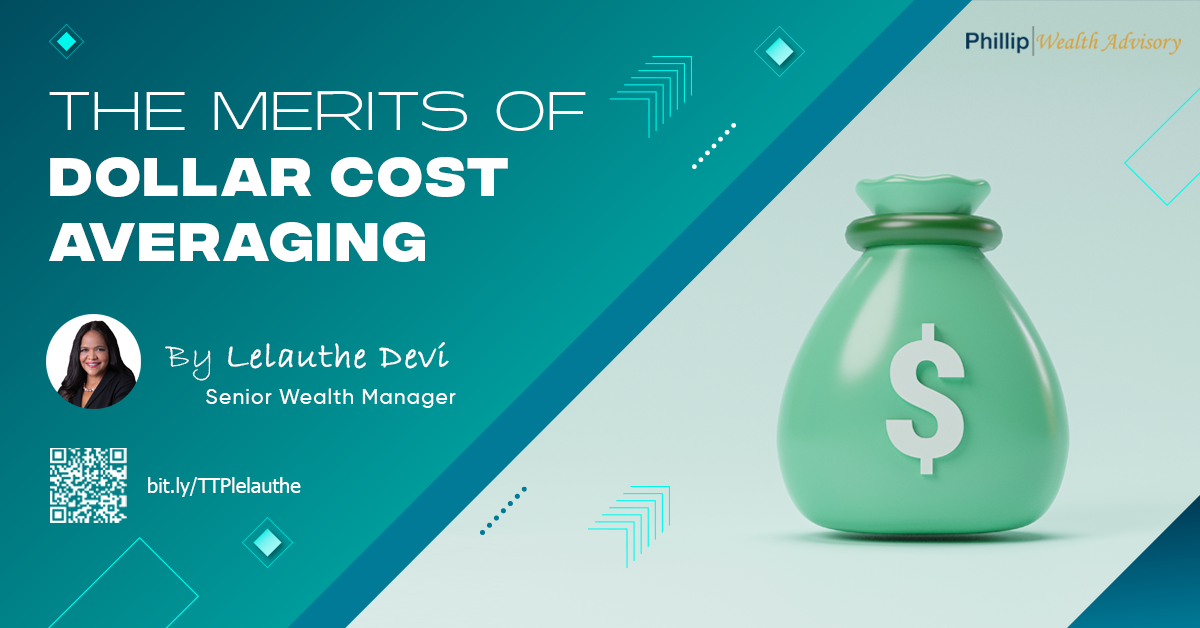#### The Merits of Dollar Cost Averaging

Published on Sep 15, 2023 48

Have you ever seen your colleagues, friends or family members on the phone with their...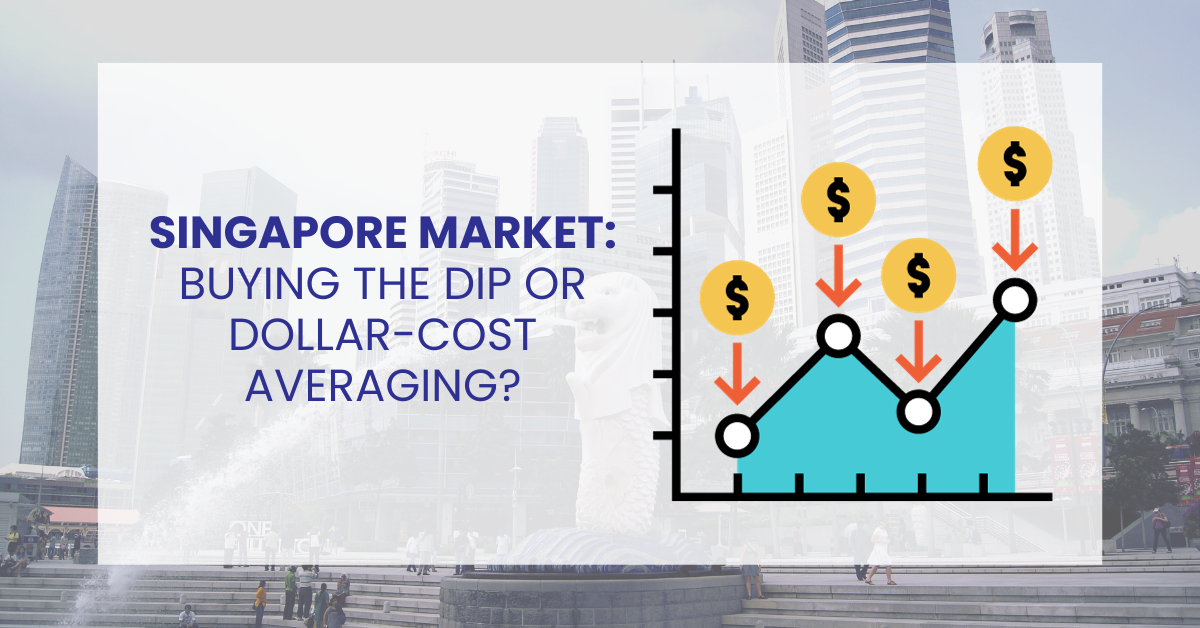#### Singapore Market: Buy the Dip or Dollar-Cost Averaging?

Published on Sep 14, 2023 46

To the uninitiated, investing in the stock market can be deemed exhilarating and challenging. The...#### What are covered calls and why are they so popular?

Published on Sep 12, 2023 391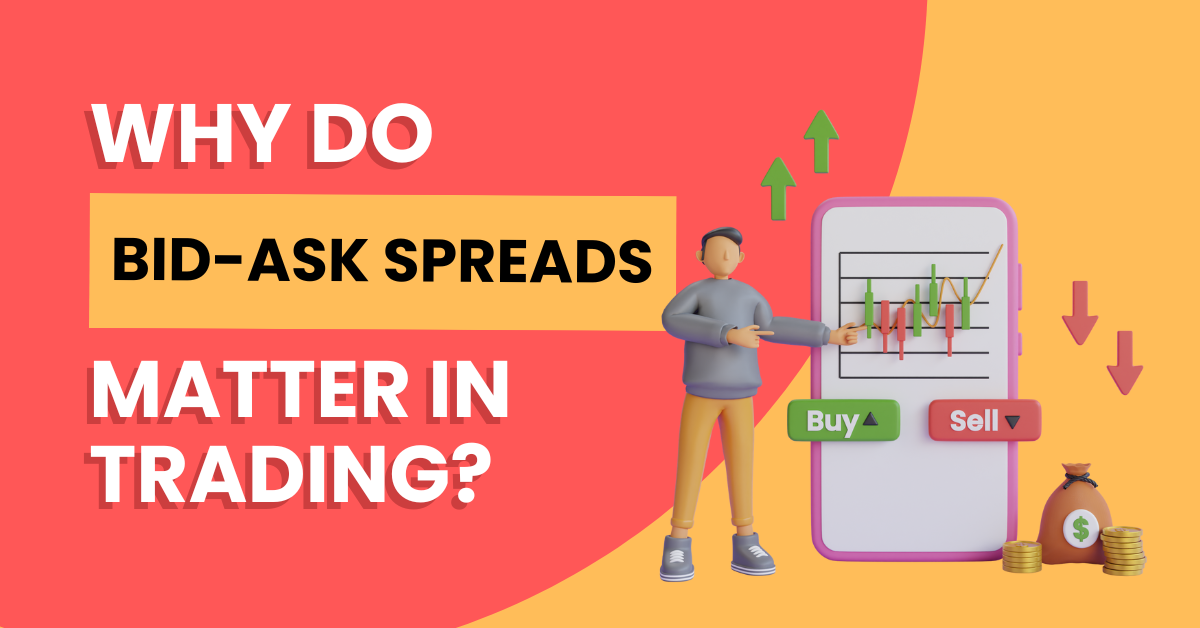Published on Sep 11, 2023 30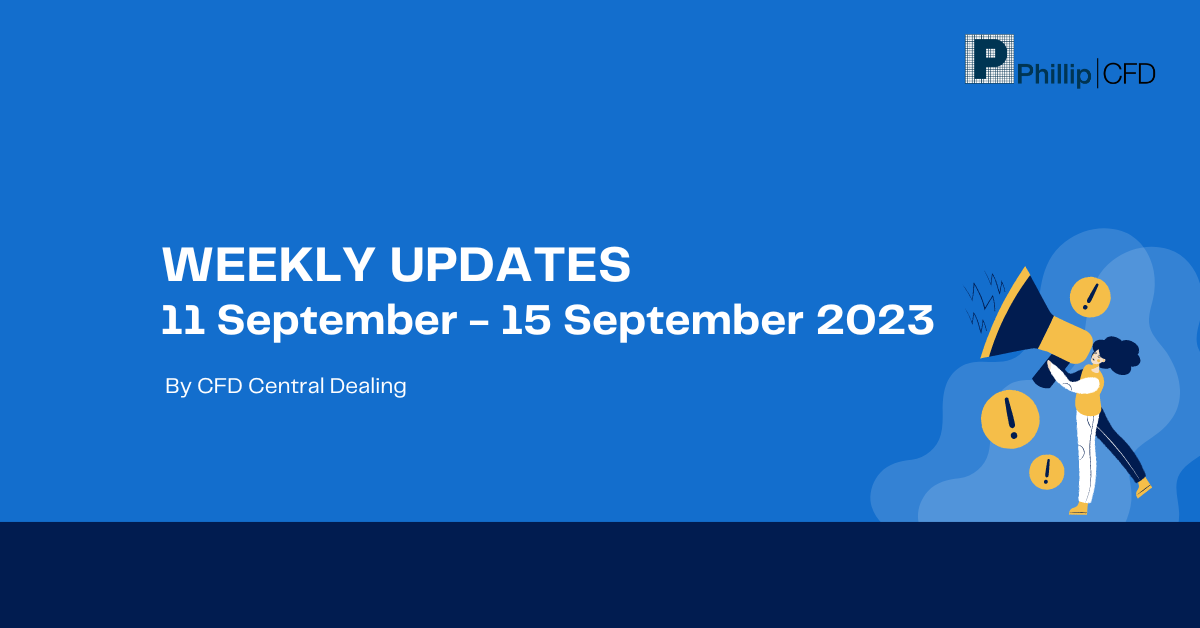#### Weekly Updates 11/9/23 – 15/9/23

Published on Sep 11, 2023 13

This weekly update is designed to help you stay informed and relate economic and company...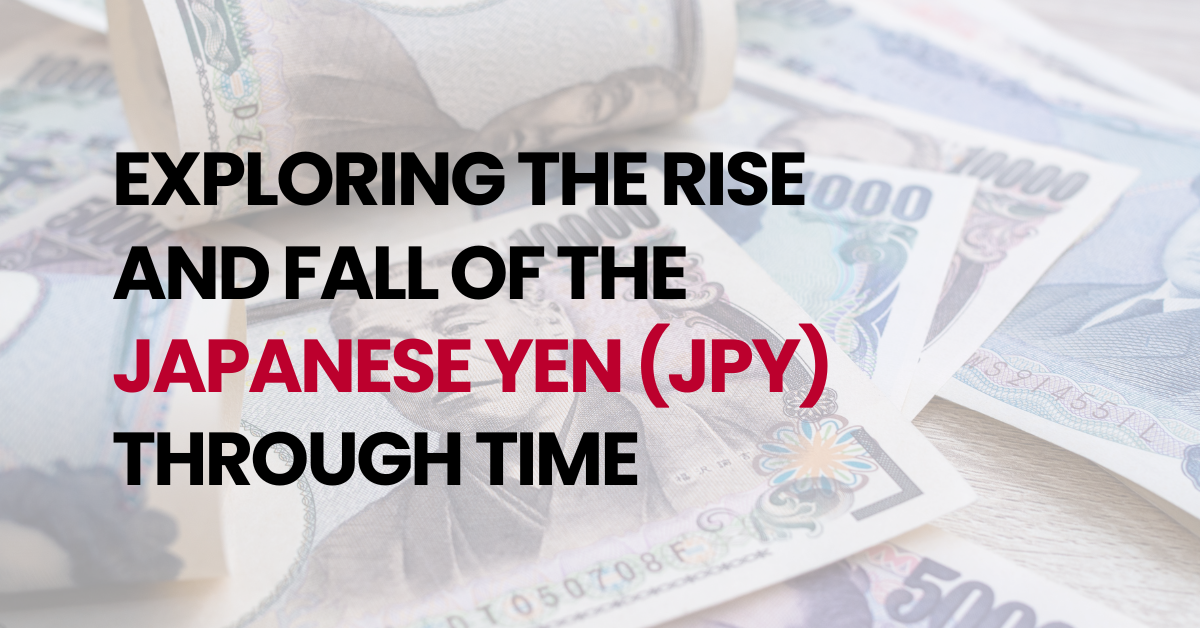#### Exploring the Rise and Fall of the Japanese Yen (JPY) Through Time

Published on Sep 5, 2023 80

The Japanese Yen (JPY) has long been considered a safe-haven currency due to Japan’s strong...